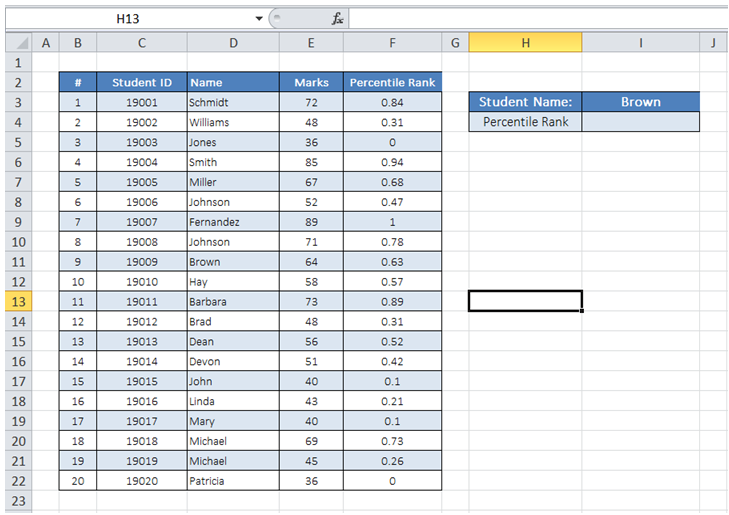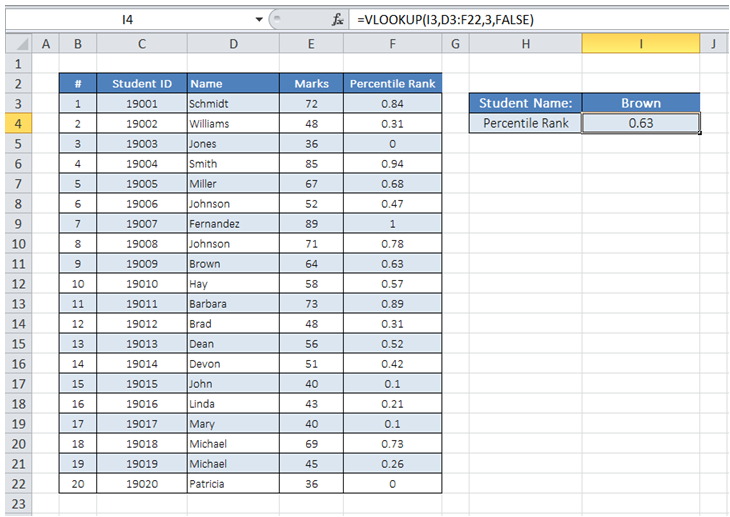## Retrieve data from specific table column in Excel

For example, I want to enter a Student Name in cell I3 and get the corresponding Percentile Rank information pulled from the table in cell I4.To do it in Excel, here is the answer:

a) Enter the formula =VLOOKUP(I3,D3:F22,3,FALSE) in cell I4 where Percentile Rank for the student selected is required.

The first argument "I3" has the Lookup value - the Student name whose Percentile Rank is to be pulled from the Table.

The second argument "D3:F22" is the Table data range with the data of interest. With VLOOKUP function, the first column in Data Range should be the column that has the Look Up value (Student name in this case).

The 3rd argument "3' is the column number in the Table data range from which the result should be pulled from.

The 4th argument of "FALSE" sets up the function to seek an exact match to the value entered in cell I3.